Get instant live expert help with Excel or Google Sheets“My Excelchat expert helped me in less than 20 minutes, saving me what would have been 5 hours of work!”

#### Post your problem and you'll get expert help in seconds

Your message must be at least 40 characters
Our professional experts are available now. Your privacy is guaranteed.

# Excel DECIMAL FunctionRead time: 20 minutes

Numbers can be represented in a base from 2 to 36.  While working with Excel, we are able to convert a number from any base to a base of 10.  This step by step tutorial will assist all levels of Excel users in converting a number to its decimal value by using the DECIMAL function.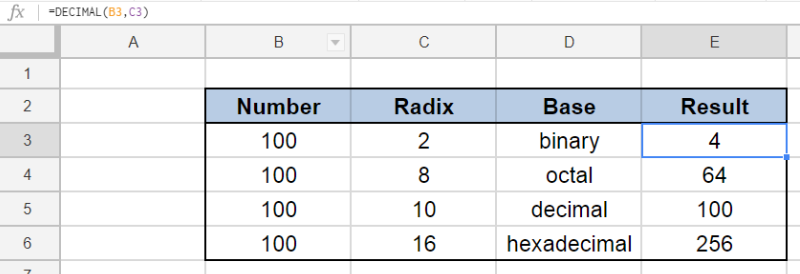Figure 1. Final result: Excel DECIMAL function

## Syntax of DECIMAL Function

DECIMAL converts any value into its decimal value; converts a value in any base to a base of 10

`=DECIMAL(number,radix)`

• number – the number we want to convert to its decimal value
• radix – the base of the value we want to convert to decimal; the base can be an integer from 2 to 36

Below table shows the alphanumeric characters for some common bases used in Excel: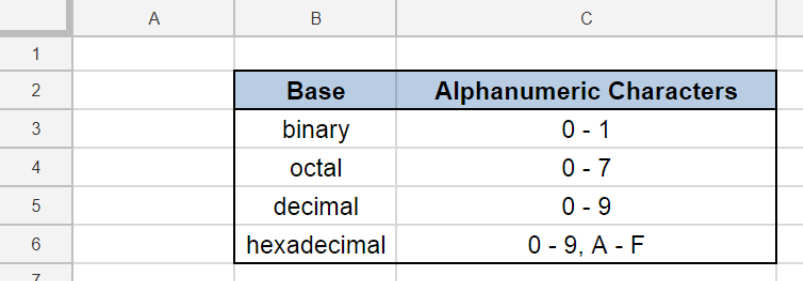Figure 2. Base and corresponding alphanumeric characters

## Setting up our Data

Our data consists of four columns: Number (column B), Radix (column C), Base (column D) and Result (column E).  We want to convert the number “100” in four different bases (2, 8, 10 and 16) into its decimal value.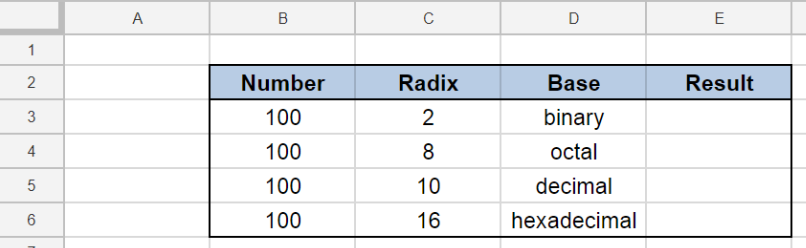Figure 3. Sample data to convert to decimal value

## Convert to decimal value

We want to determine the decimal value of the number “100” in different bases by using the DECIMAL function.  Let us follow these steps:

Step 1.  Select cell E3

Step 2.  Enter the formula: `=DECIMAL(B3,C3)`

Step 3.  Press ENTER

Step 4:  Copy the formula in cell E3 to cells E4:E6 by clicking the “+” icon at the bottom-right corner of cell E3 and dragging it down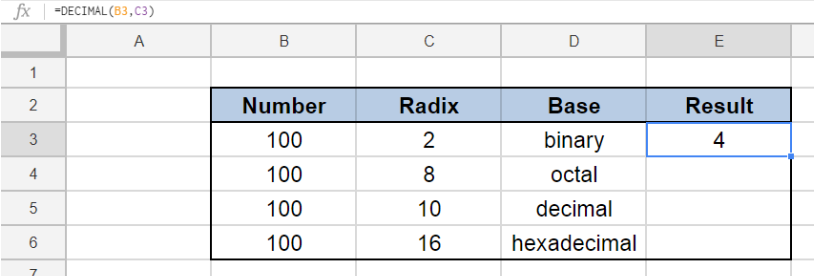Figure 4.  Using the DECIMAL function to convert to decimal value

The value in B3 100 is a binary number with a base of 2.  Its resulting decimal value in E3 is “4”.

For the succeeding examples, the numbers are converted to their corresponding decimal values in cells E4to E6.  Note that different bases for the same number, in this case “100”, return different decimal values.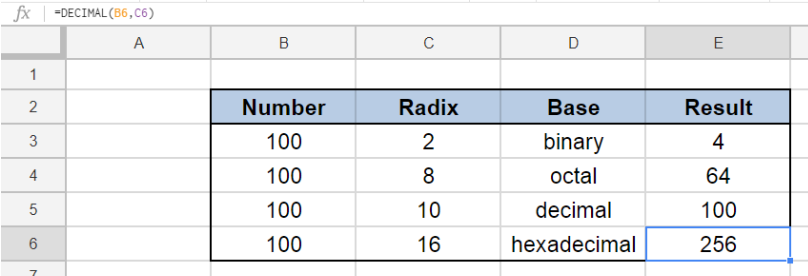Figure 5.  DECIMAL returns different results for different bases

The DECIMAL function can be used to convert any number with a base from 2 to 36 into its decimal value.

Note, however, that the DECIMAL function returns a #NUM! error when the value we want to convert contains characters that are not valid representations of the given base.  In our example below, “22” is not a valid binary representation because binary numbers contain only the digits 0 and 1.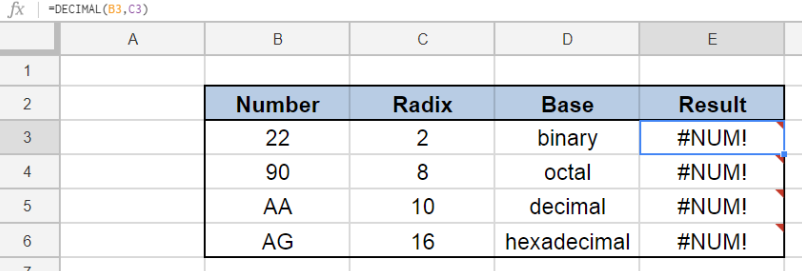Figure 6.  DECIMAL returns error value for invalid characters

Most of the time, the problem you will need to solve will be more complex than a simple application of a formula or function. If you want to save hours of research and frustration, try our live Excelchat service! Our Excel Experts are available 24/7 to answer any Excel question you may have. We guarantee a connection within 30 seconds and a customized solution within 20 minutes.

### Did this post not answer your question? Get a solution from connecting with the expert.Another blog reader asked this question today on Excelchat:## Subscribe to Excelchat.coAnother blog reader asked this question today on Excelchat: